Activate JavaScript to enable computations!

# Pulsed Power Toolbox

This is the start of a collection of Pulsed Power related formulas and calculators that I found usefull. However, whenever I could find resources on the internet already, I tried to refer to them rather than to re-invent the wheel. Consequently you might also find some of the links provided helpful.

## Formulas for the Calculation of Common Capacitances*)

(unless otherwise noted use SI-units in all presented formulas)

*) Of course no guarantee is given for the accuracy of the listed formulaes or the calculators although I tried my best to select the formulas carefully from the literature. Please let me know when you find any mistakes.

capacitance

formula

example

parallel plates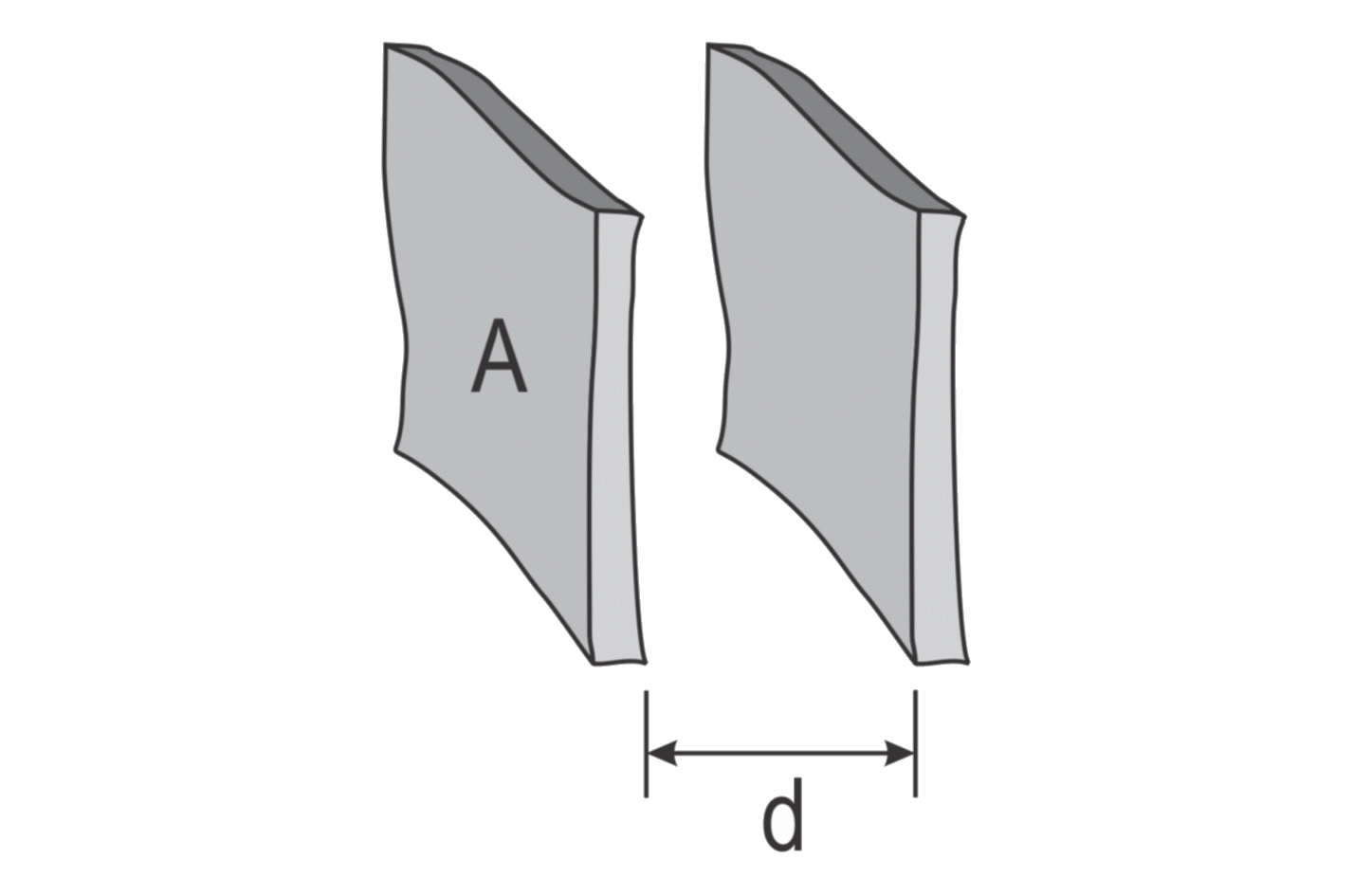A >> d2: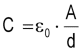A = 2 cm2 d = 0.2 cm C = 88.5 fF

long parallel strips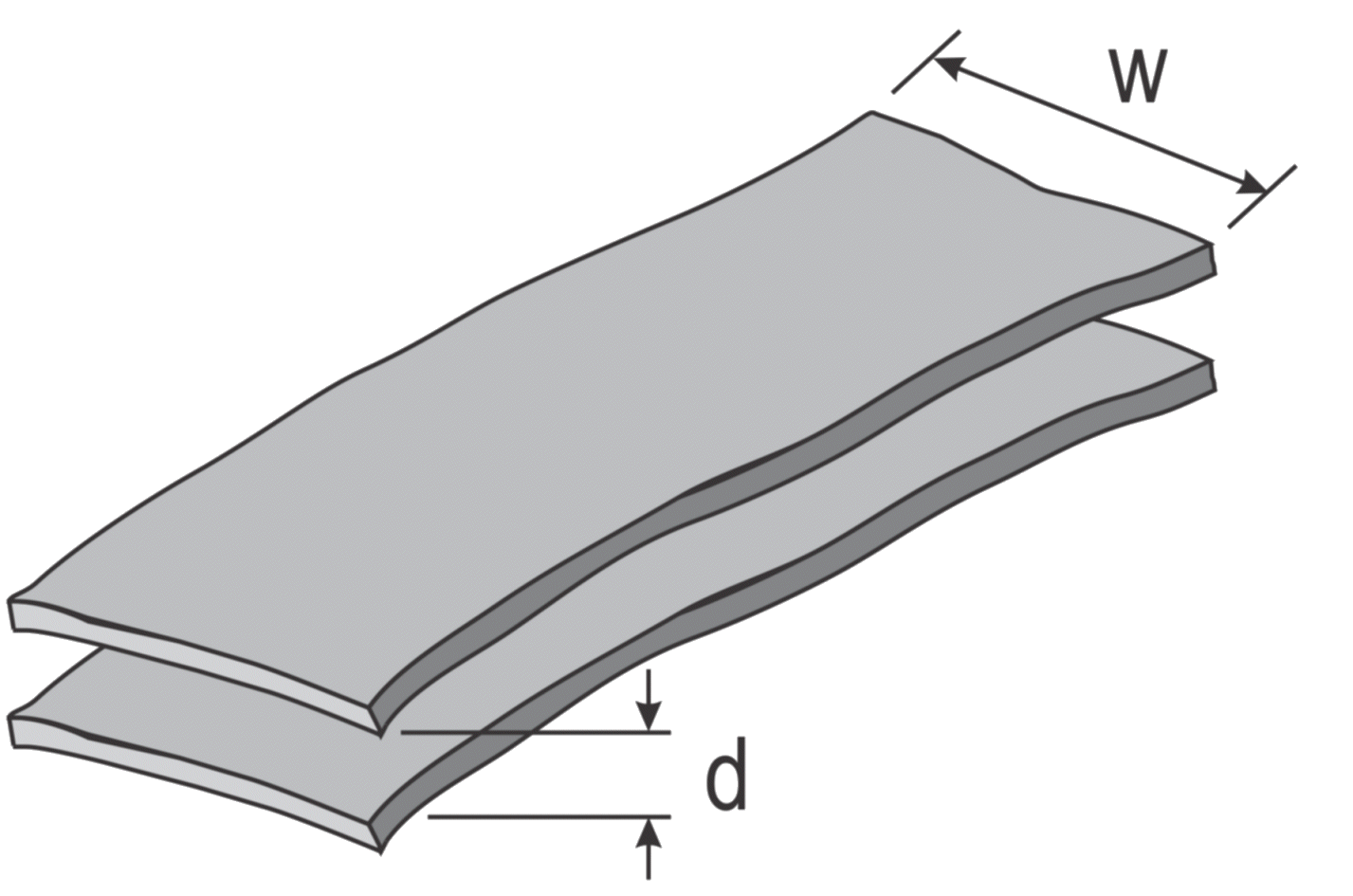For w/d < 1: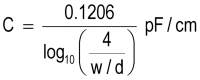For w/d > 1: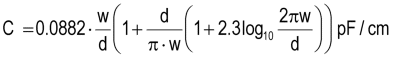w = mm d = mm pF/cm

isolated disk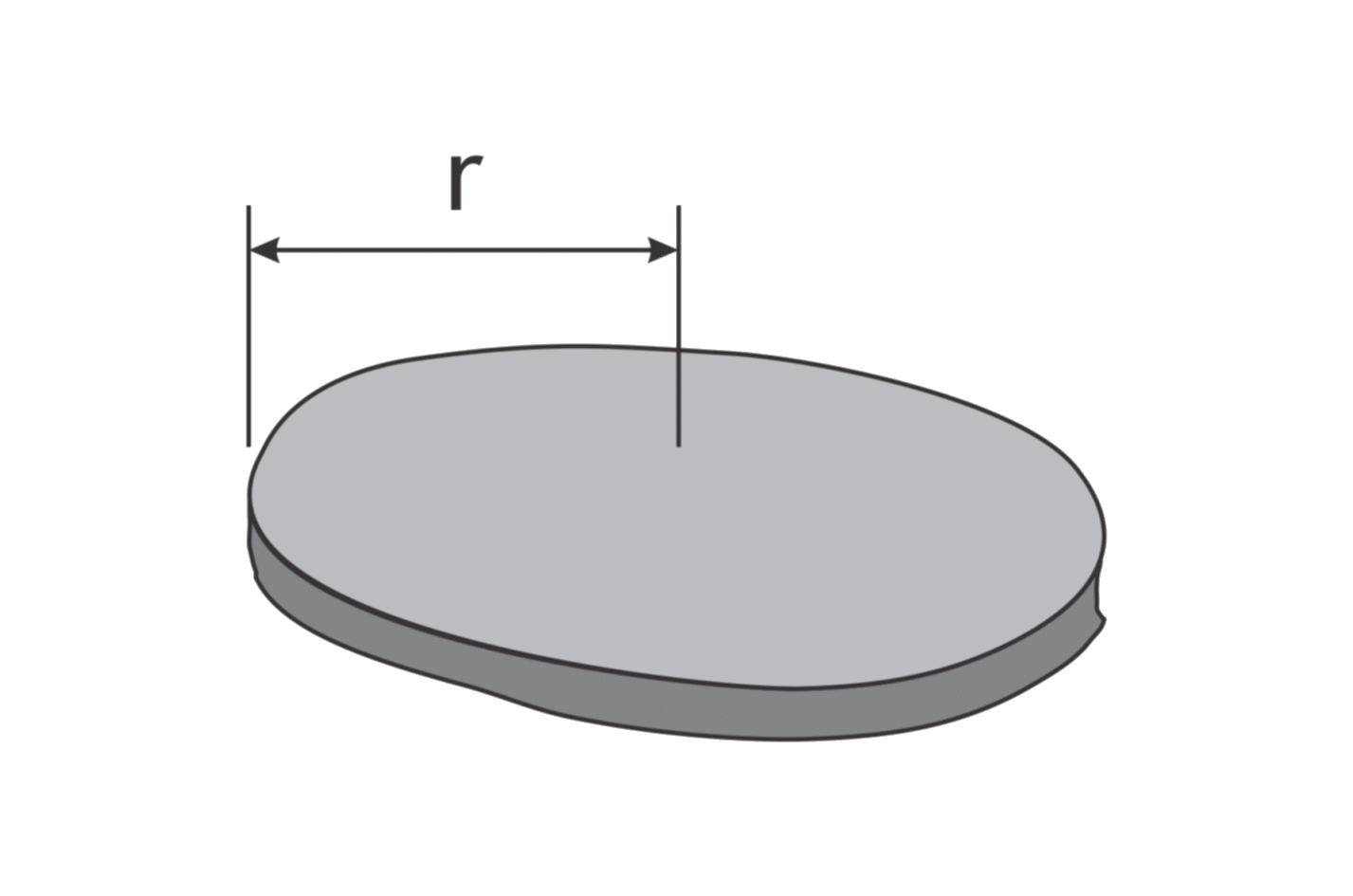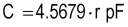r = cm pF

two spheres of equal size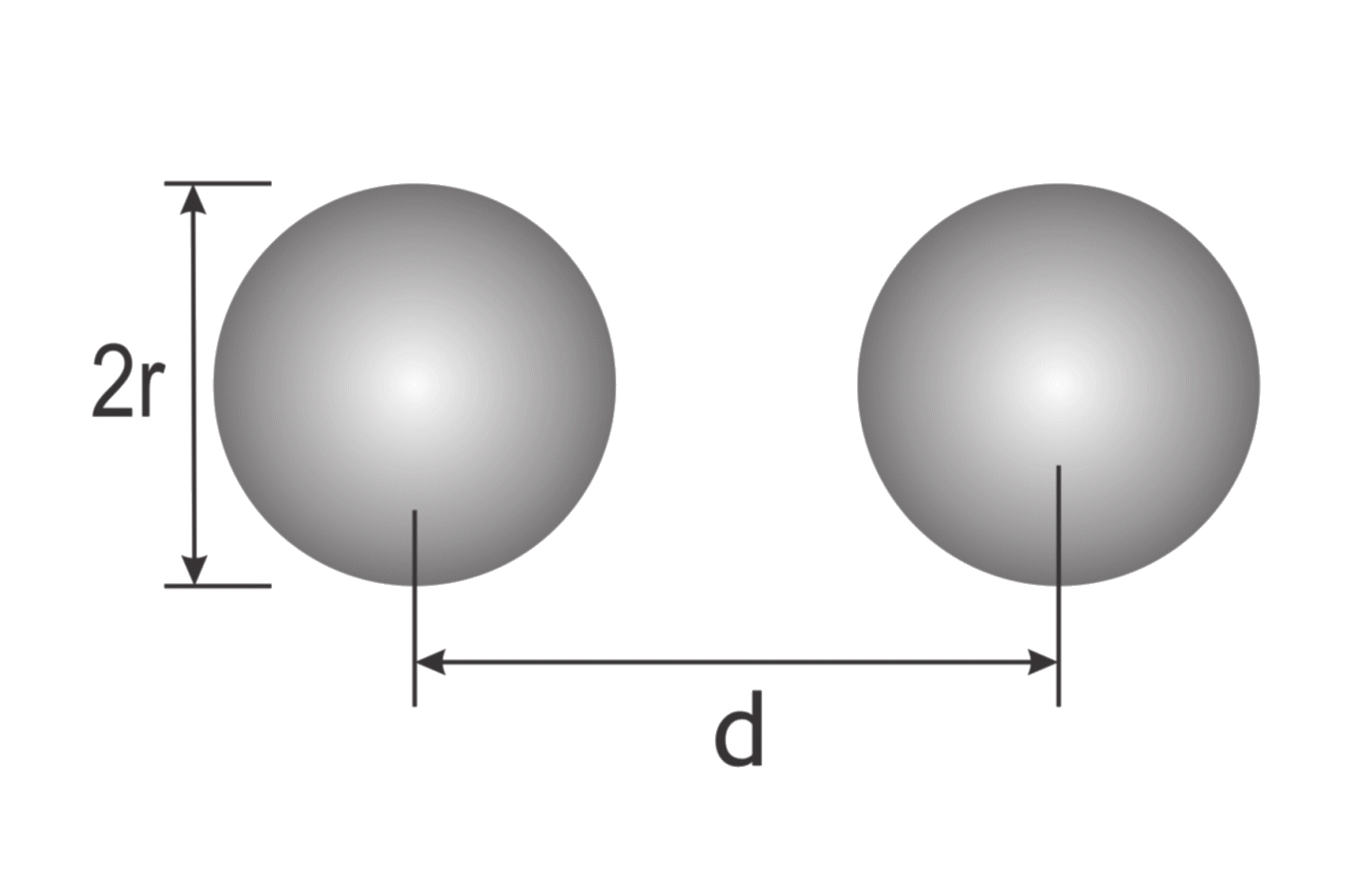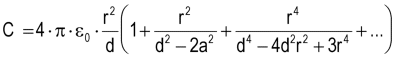r = cm d = cm pF

isolated sphere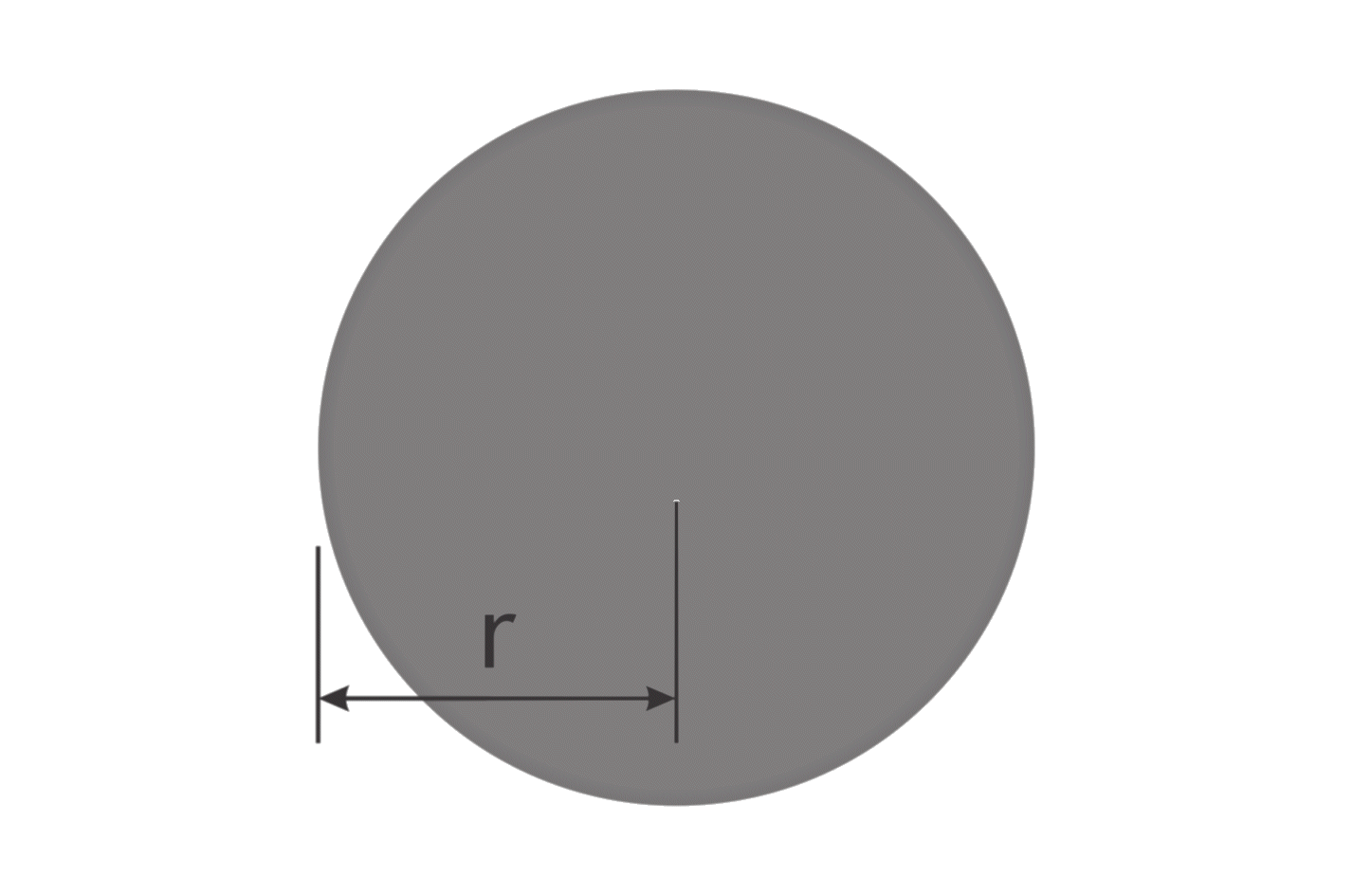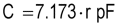r = cm pF

concentric spheres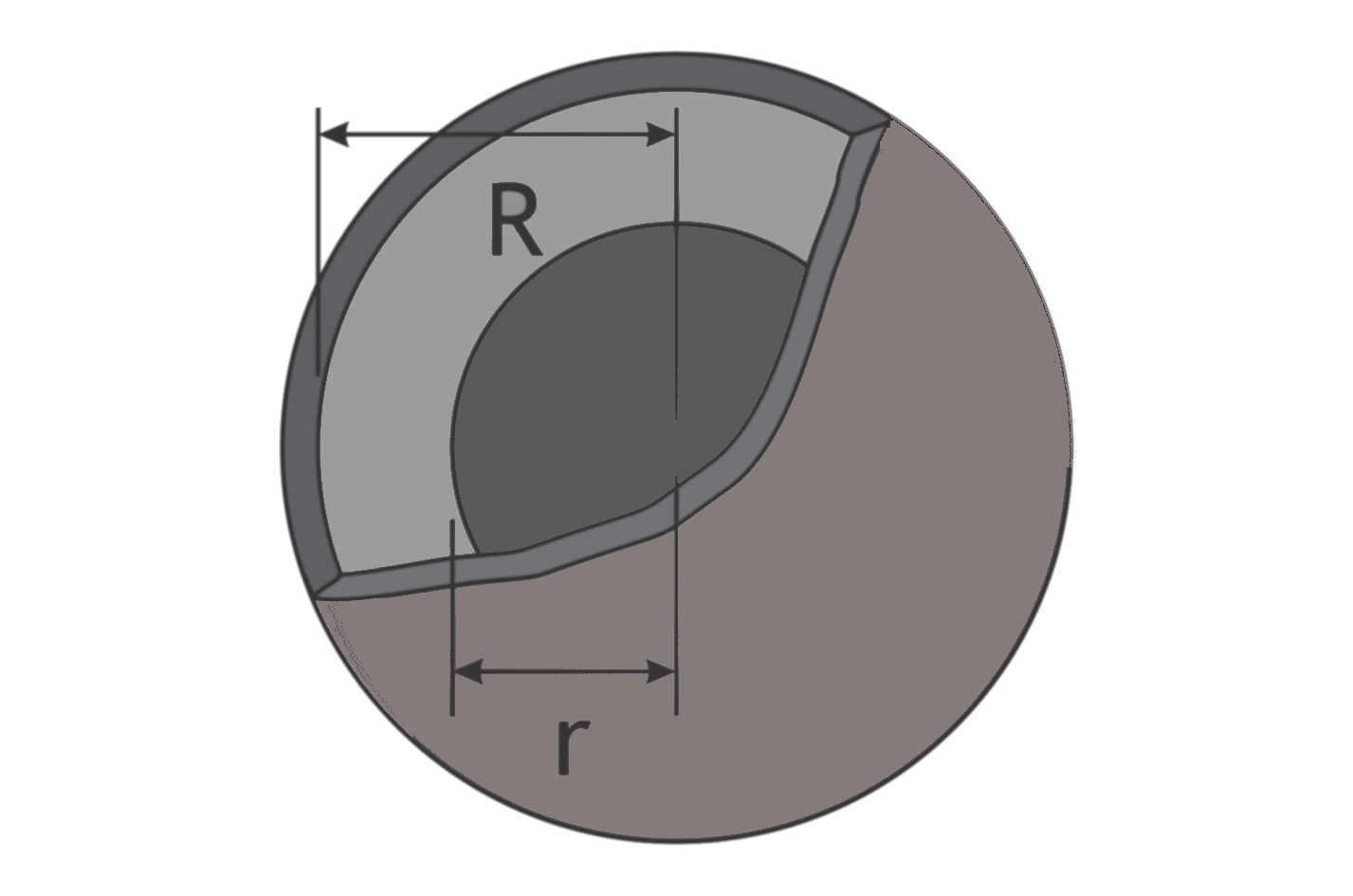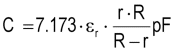εr: relative permittivity
of dielectric between spheres

 εr r = cm R = cm pF

coaxial cable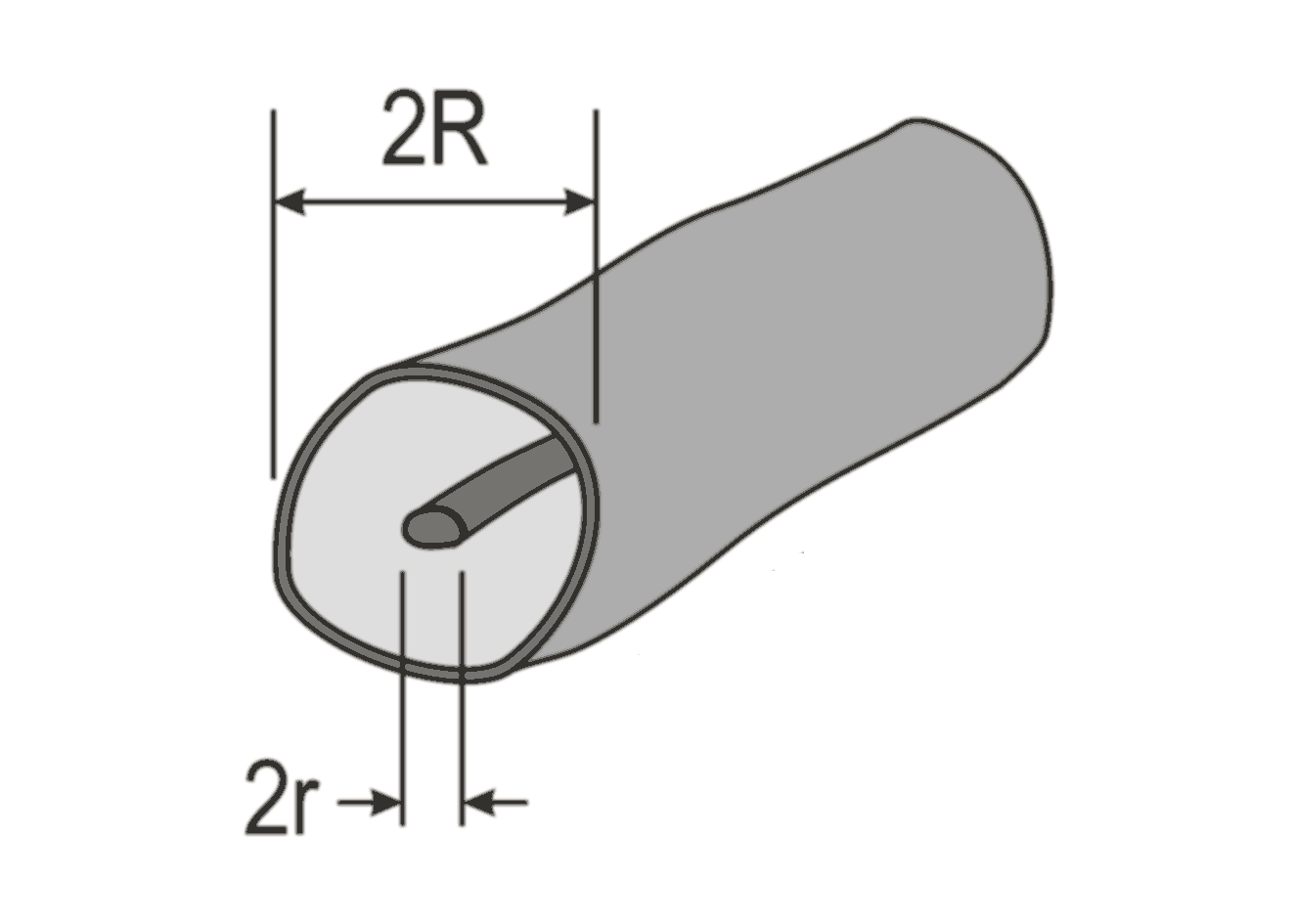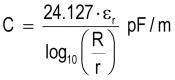εr: relative permittivity
of dielectric between conductors

 εr = R = mm r = mm pF/m

infinetly long, thin wire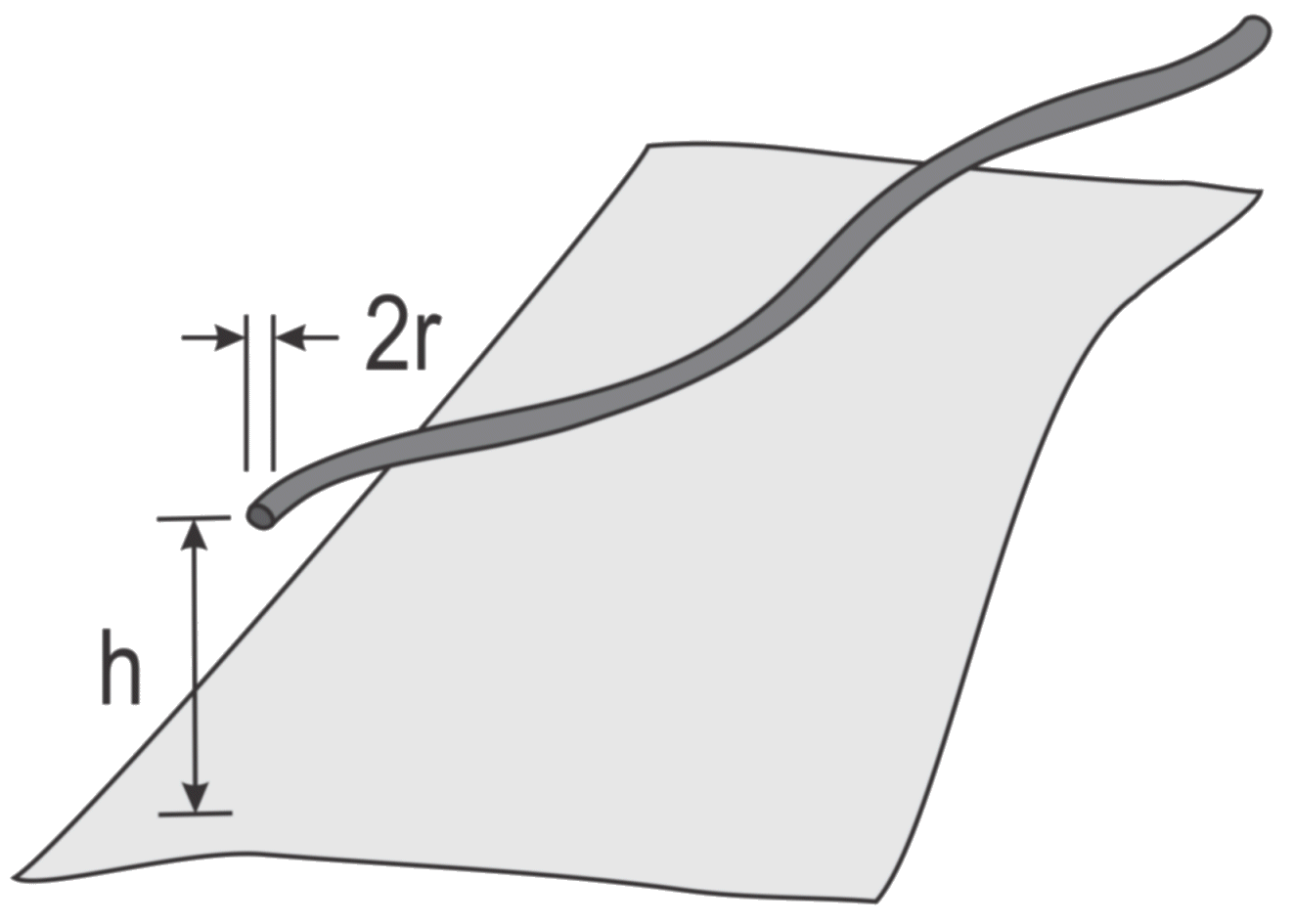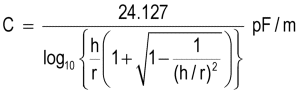h = cm r = mm pF/m

For h>>r: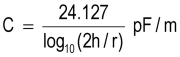h = 100 cm r = 0.5 mm C = 6.7 pF/m

thin wire of finite length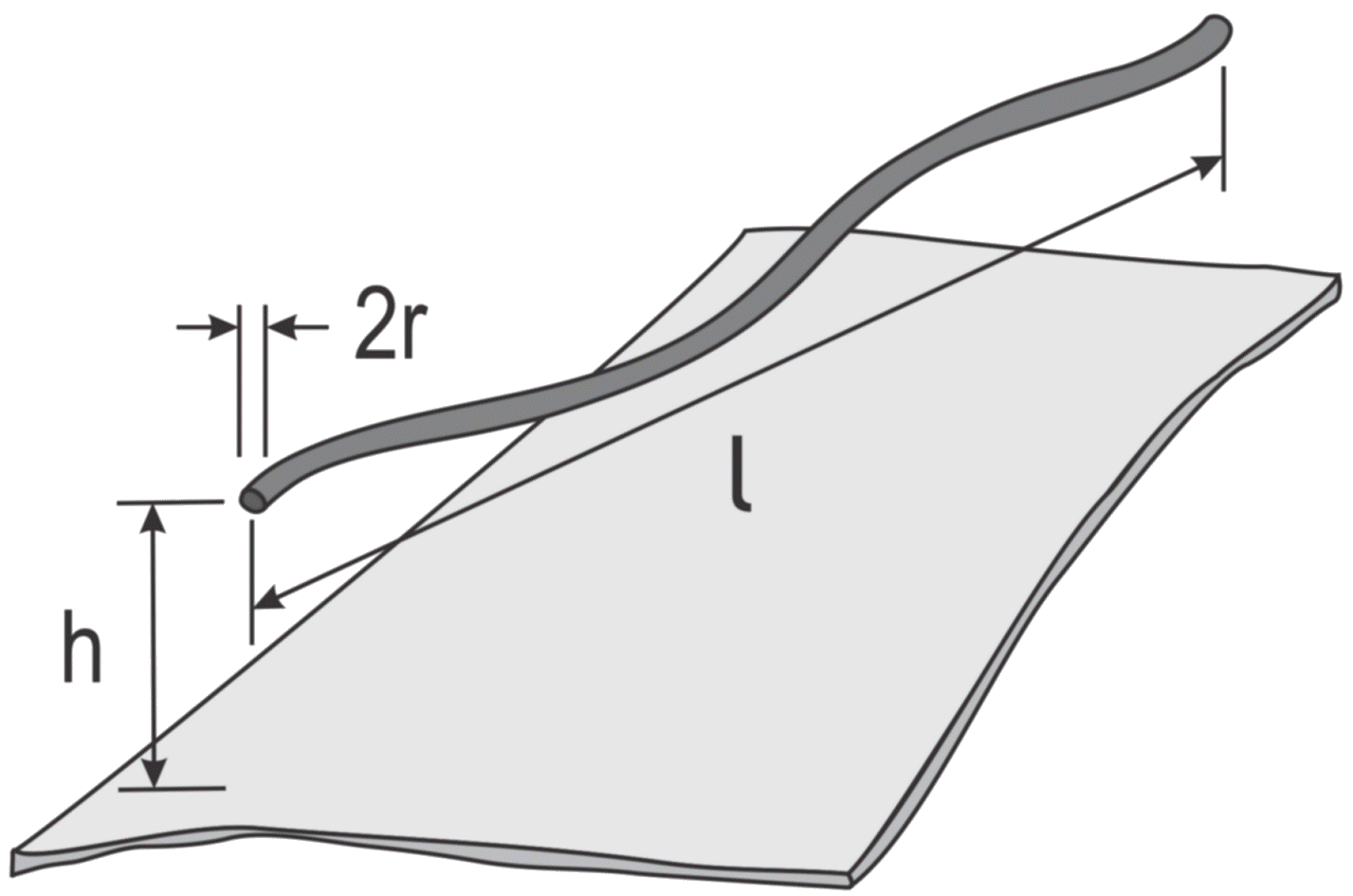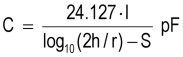S-factor is computed automatically

(argument 2h/l or l/2h dpending on which is less than unity)

 h = cm r = mm l = cm pF

prallel wires over ground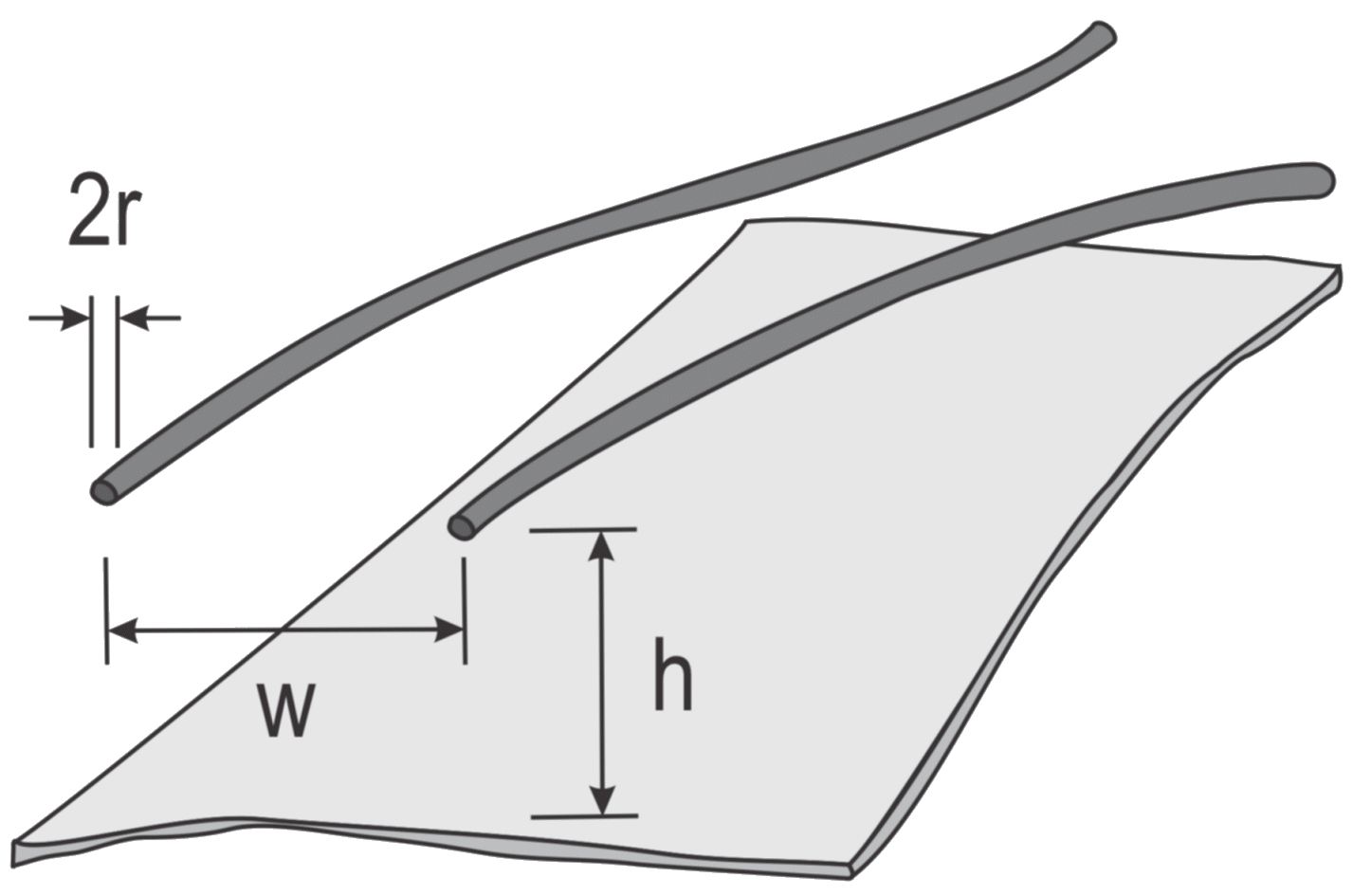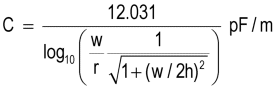r = mm w = cm h = cm pF/m

prallel wires remote from ground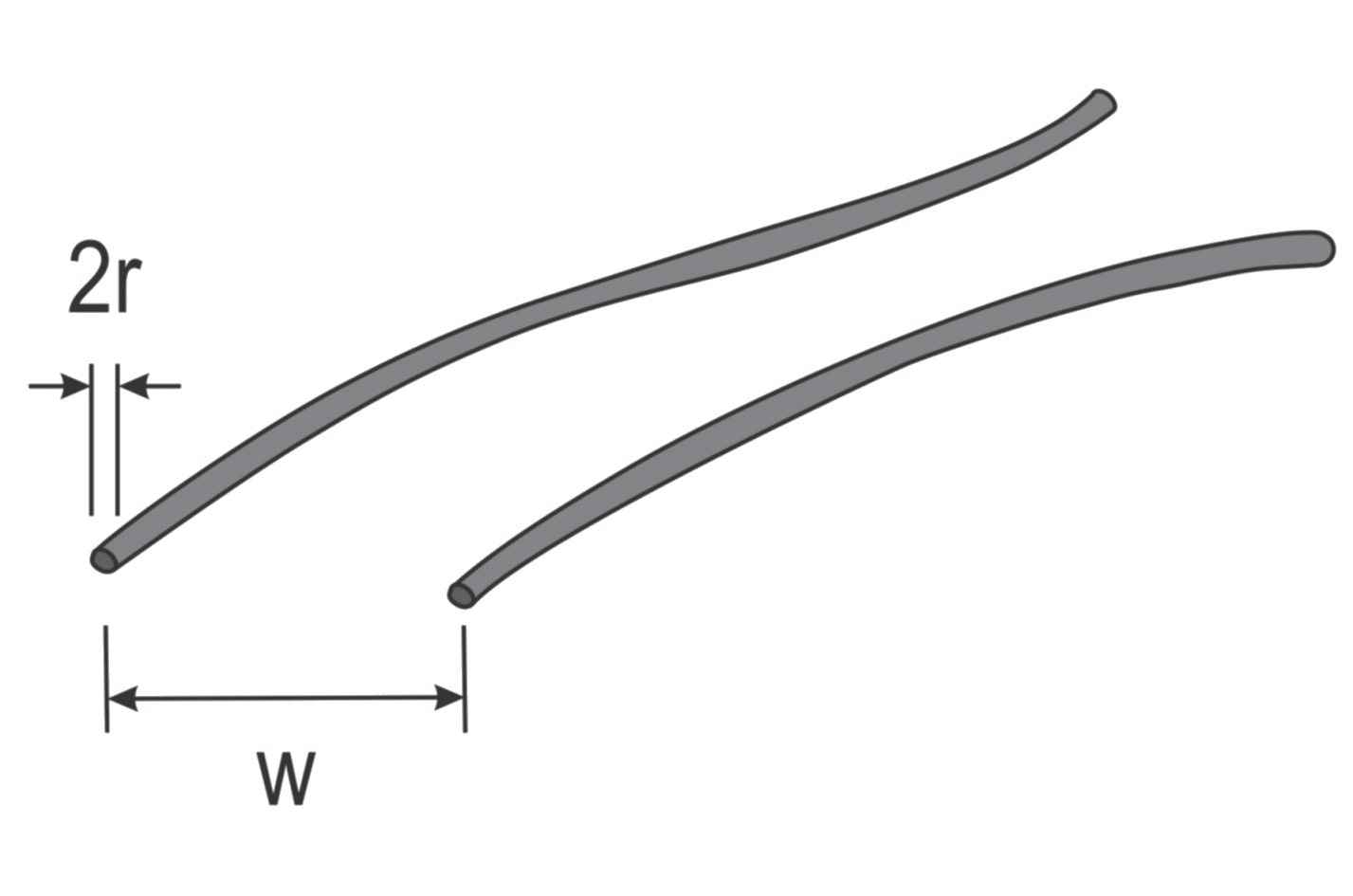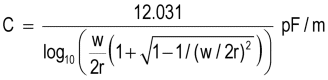r = mm w = cm pF/m

parallel wires of finite length
above ground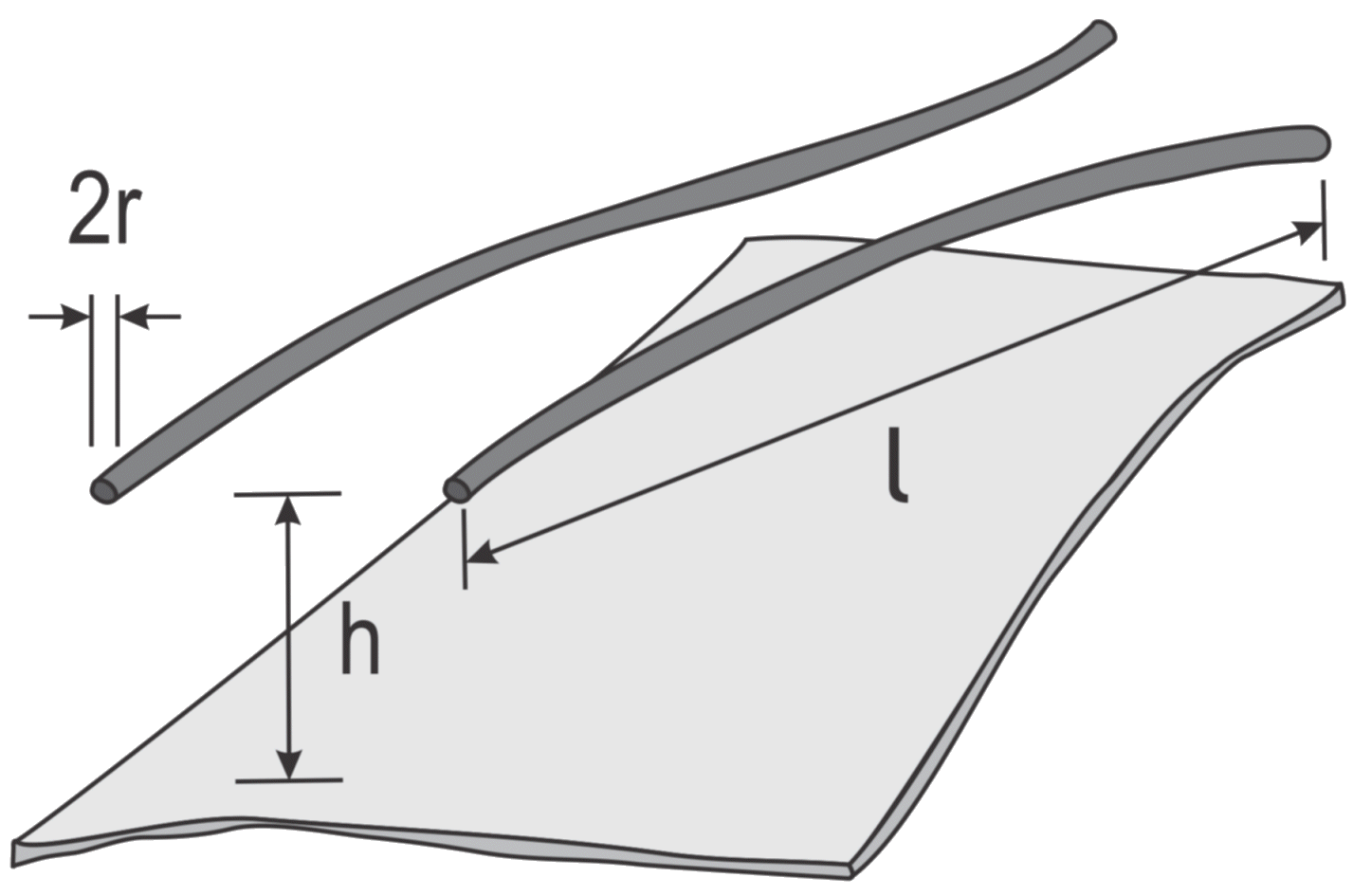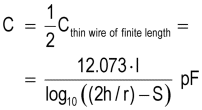vertical aligned wires
parallel to ground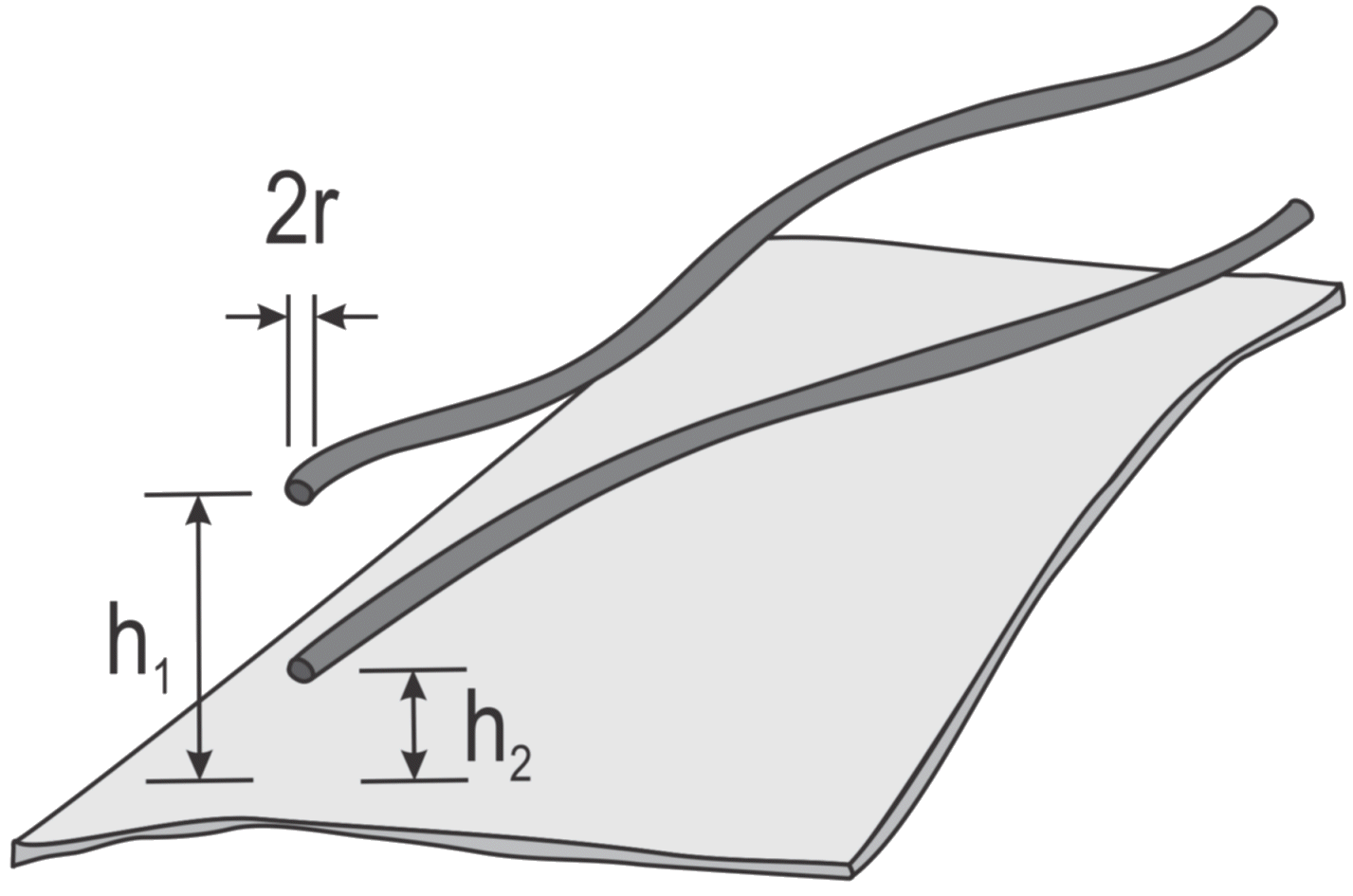calculated like
"parallel wires over ground"
with h = (h1+h2)/2

single layer solenoid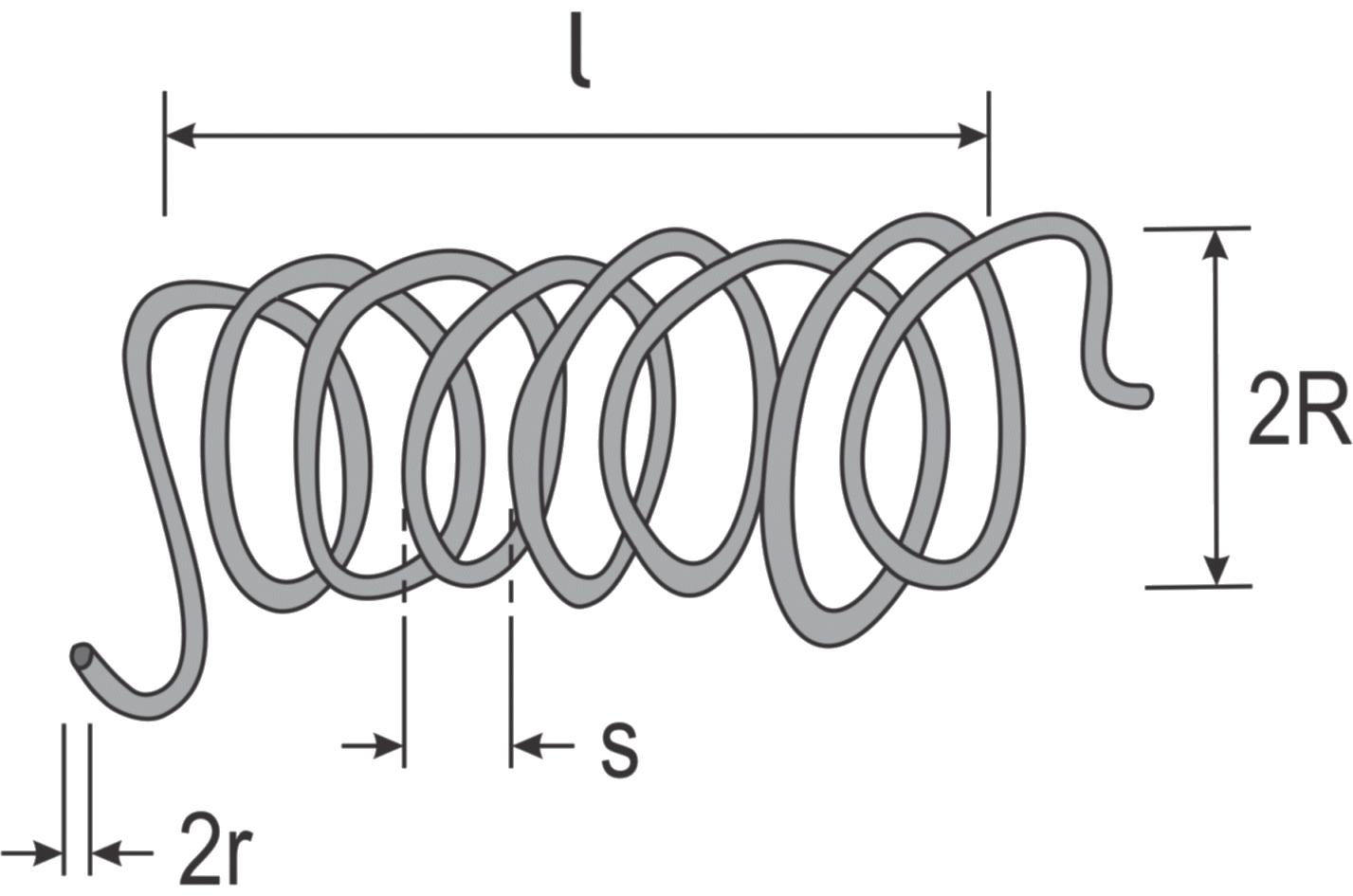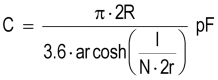N: number of turns

 R = mm r = mm l = mm N = pF

 References:     1) F.E. Terman, Radio Engineers' Handbook, McGraw-Hill, New York, 1943.